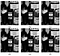# Review — Blind Image Blur Estimation via Deep Learning (Blur Classification)

## Gaussian, Motion & Defocus Blur Classification

In this story, Blind Image Blur Estimation via Deep Learning, by Nanjing University of Information Science and Technology, The University of Sheffield, and Northumbria University, is reviewed. In this paper:

# Outline

1. DNN & GRNN Framework
2. Deep Neural Network (DNN)
3. General Regression Neural Network (GRNN)
4. Experimental Results

# 1. DNN & GRNN Framework

## 1.1. Framework

• GRNN is the blur PSF parameter estimation, which has different output labels for each blur type. P1, P2, and P3 are the estimated parameters.

## 1.2.1. Gaussian Blur

• In many applications, such as satellite imaging, Gaussian blur can be used to model the PSF of the atmospheric turbulence:

## 1.2.2. Motion Blur

• Another blur is caused by linear motion of the camera, which is called motion blur:

## 1.2.3. Defocus Blur

• The third blur is the defocus blur, which can be modeled as a cylinder function:

# 2. Deep Neural Network (DNN)

## 2.1. Pretraining

1. The input layer is trained in the first RBM as the visible layer. Then, a representation of the input blurred sample is obtained for further hidden layers.
2. The next layer is trained as an RBM by greedy layer-wise information reconstruction. The training process of RBM is to update weights between two adjacent layers and the biases of each layer.
3. Repeat the first and second steps until the parameters in all layers (visible and all hidden layers) are learned.

## 2.2. Fine-tuning

• In the supervised learning part, the above trained parameters are used for initializing the weights in the DNN.
• The goal for the optimization process is to minimize the backpropagation error derivatives, i.e. cross-entropy loss:

## 2.3. Some Details

• The output of this stage is 3 labels: the Gaussian blur, the motion blur and the defocus blur.
• The size of samples is 32 × 32.
• The input visible layer has 1024 nodes, and the output layer has 3 nodes.
• The whole architecture is: 1024 → 500 →30 → 10 → 3.
• With the label information from DNN, the classified blur vectors will be used in the second stage (GRNN) for blur parameter estimation.

# 3. General Regression Neural Network (GRNN)

• The general regression neural network is considered to be a generalization of both Radial Basis Function Networks (RBFN) and Probabilistic Neural Networks (PNN).
• It is composed of an input layer, a hidden layer, “unnormalized” output units, a summation unit, and normalized outputs.
• Assume that the training vectors can be represented as X and the training targets are Y.
• In the pattern layer, each hidden unit is corresponding to an input sample.
• From the pattern layer to the summation layer, each weight is the target for the input sample. The summation units can be denoted as:

# 4. Experimental Results

## 4.1. Dataset

• Training Datasets: The Oxford image classification dataset,2 and the Caltech 101 dataset are chosen to be the training sets. 5000 images are randomly selected from each of them. The size of the training samples is 32 × 32.
• Each training sample has two labels: one is its blur type (the values are 1, 2, or 3) and the other one is its blur parameter.
• At last, there are 36000 training samples, 12000 of them are degraded by Gaussian PSF, 12000 of them are degraded by the PSF of motion blur, and the rest are degraded by the defocus PSF.
• Testing Datasets: Berkeley segmentation dataset (200 images), Pascal VOC 2007: 500 images are randomly selected.
• 6000 testing samples are chosen from each of them according to the same procedure as the training set.

## 4.2. Classification Results

• The classification rate is used for evaluating the performance:

## 4.3. Regression Results

• Then, quantitative metric is used to evaluate the deblurred image quality, i.e. some Image Quality Assessment (IQA) approaches.
• (The paper does not mention what deblurring algorithm is used for the above table.)Comparison of the deblurred results of images corrupted by motion blur with length 10 and angle 45. (a) Ground truth. (b) The blurred image. (c) CNN. (d) Levin et al. . (e) Cho and Lee . (f) GRNN.

## Reference

[2016 TIP] [DNN+GRNN]
Blind Image Blur Estimation via Deep Learning

## Blur Classification

2014 [Tiwari IJMECS’14] 2016 [DNN+GRNN] 2017 [Tiwari IJEEI’17] [SFA] 2019 [SFA & SFGN] 2020 [Gueraichi SAI’20] [Szandała SSCI’20] [Tiwari IJISMD’20]